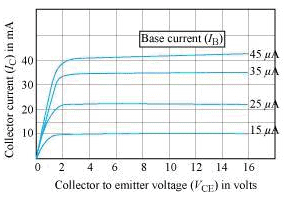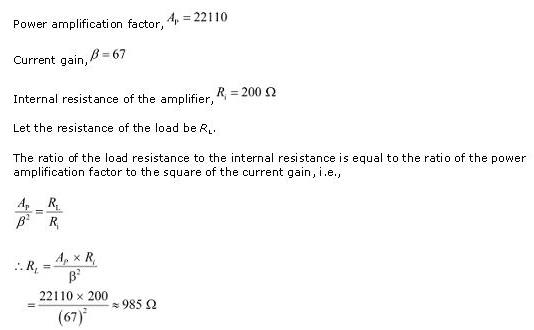Courses

# Test: Applications Of Transistors

## 10 Questions MCQ Test Physics For JEE | Test: Applications Of Transistors

Description
This mock test of Test: Applications Of Transistors for JEE helps you for every JEE entrance exam. This contains 10 Multiple Choice Questions for JEE Test: Applications Of Transistors (mcq) to study with solutions a complete question bank. The solved questions answers in this Test: Applications Of Transistors quiz give you a good mix of easy questions and tough questions. JEE students definitely take this Test: Applications Of Transistors exercise for a better result in the exam. You can find other Test: Applications Of Transistors extra questions, long questions & short questions for JEE on EduRev as well by searching above.
QUESTION: 1

### Which of the following is not an ac parameter of a transistor?

Solution:

Input resistance, Output resistance and Current amplification factor are ac parameters of a transistor.

QUESTION: 2

### What is the voltage gain in a common emitter, where input resistance is 3Ω and the load resistance is 24Ω, β = 0.6​

Solution:

Voltage gain   =β× (Rc​​/Rb​)
Or,   Voltage gain =0.6×(24/3)​=4.8

QUESTION: 3

### An oscillator is nothing but an amplifier with:

Solution:

An oscillator is an electronic circuit that converts DC from a power supply to an AC signal. An oscillator produces a periodic and oscillating signal. An oscillator is like an amplifier that gives positive feedback to the circuit. Positive feedback means that a portion of output is combined in phase with the input.

QUESTION: 4

The output characteristic for a common emitter transistor is shown in the given figure.Use the graph to find out the current amplification factor for the transistor when VCE = 2 V.​

Solution:
QUESTION: 5

The power amplification for a common emitter (CE) amplifier is 22110. Its current gain is 67 and internal resistance is 200 ohm. What is the value of the of the output resistance?​

Solution:QUESTION: 6

A common emitter (CE) amplifier has a current gain of 49. The voltage drop across the collector terminal is 1.8 V. The collector resistance is 2.2 kilo-ohm. What is the base current in the CE amplifier?​

Solution:

Given,
Current gain(B)=4g
Voltage drop across terminal
Collector=1.8v
Collector resistance=2.2kΩ
Collector current=1.8/2.2x103
Current gain=△IC/△IB
△IB=1.8/2.2x103x4g
(D)△IB=1.67x10-5 A

QUESTION: 7

Which of the following relations correctly shows the value of current amplification factor at a constant collector-emitter voltage?

Solution:
QUESTION: 8

Transistors are useful in equipments such as hearing aids and small radio receivers because transistors :

Solution:

Transistors consume very less energy and are therefore used in these equipment because these equipment don't have space for big power source.

QUESTION: 9

The input voltage applied to a cascaded amplifier (consisting of two amplifiers) is 0.02 V and the output voltage is 6 V. The voltage gain of one of the two amplifiers is 10. What is the voltage gain of the other amplifier?​

Solution:
QUESTION: 10

In a common base transistor circuit, the current gain is 0.98. On changing emitter current by 5 mA, the change in collector current is​

Solution:

Given, That the current gain is 0.98
The change in emitter current is Ie​=5mA
Therefore, the change in collector current is Ic​=0.98×Ie​=0.98×5=4.9mA
Therefore, the correct option is C.